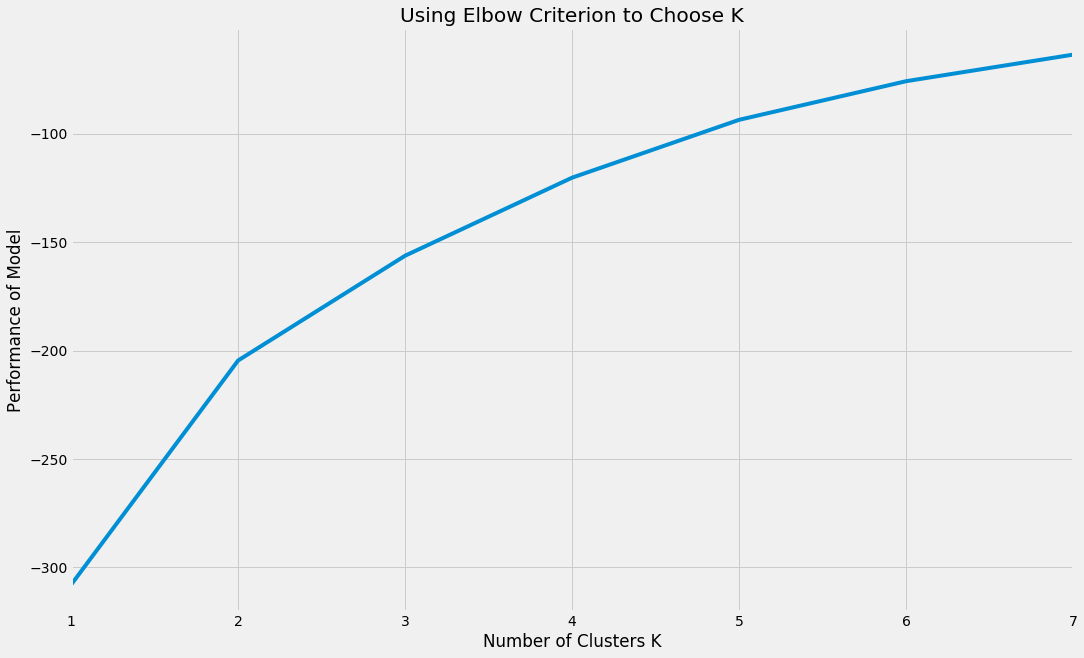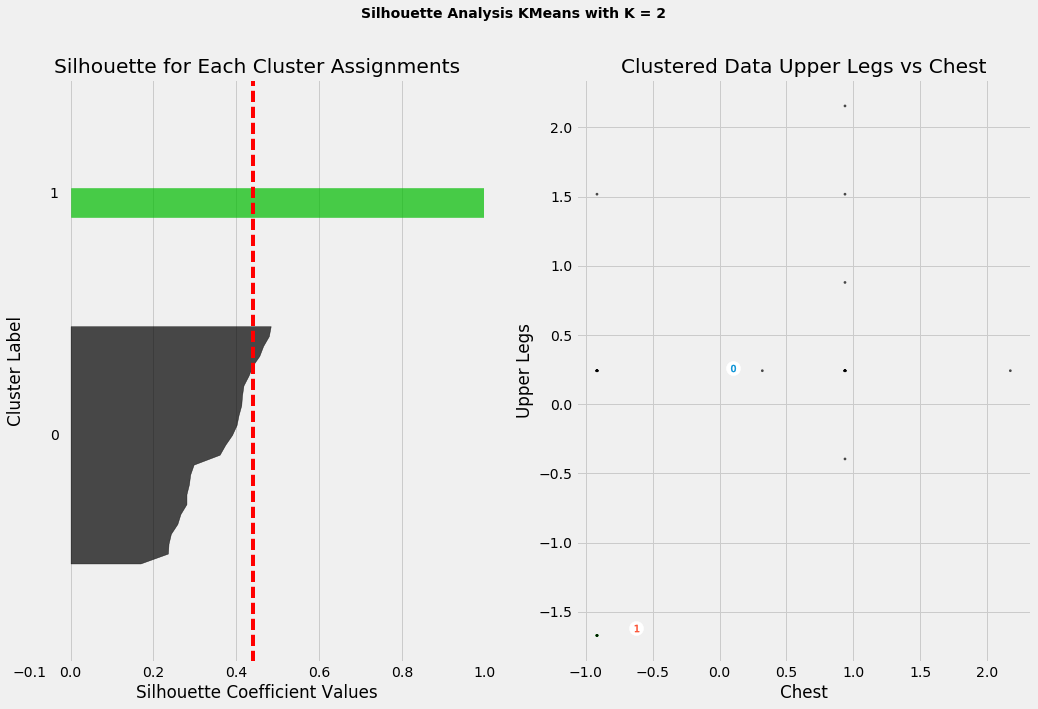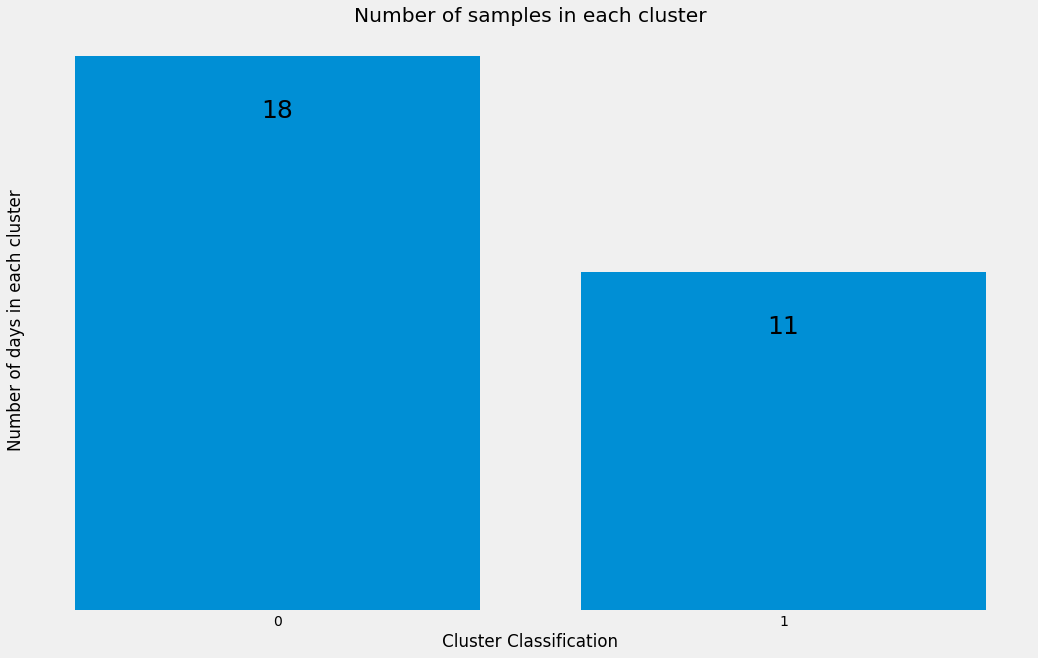# Unsupervised Machine Learning to Quantify Exercise Performance

[Note: This builds on previous work, scraping MyFitnessPal here, importing and cleaning Jefit data here, and creating a class to merge and deliver basic analysis on both here]

We’d like compare the effects of nutrition on exercise performance. Firstly, we must define what constitutes “good” performance and how we can extract it from exercise logs containing exercise names, sets, reps, weights, etc.

There are several options with their own pros and cons.

• We can consider if a new record was set, whether longest distance run, heaviest bench press, etc. This provides an incomplete picture, though; one might usually run after a weight lifting session, and achieve a new best on a day you skip the weights and simply have more energy to spend on it. Likewise, you might set a record on an exercise you normally do half-way through a workout if you instead chose to do it first.
• We could count the total volume, for example if you did 5 sets of 5 reps of an exercise with 200 lbs, that’d be 5*5*200 = 5000 lbs of volume. Unfortunately this fails to relate different exercises. For example, you might feel fantastic and spend two hours at the gym doing abs, arms, and running, but 10 sets of crunches might count as zero volume due to the lack of weight used. It’d also be too difficult to scale particular exercises, given that even within body groups there can be enormous variance (consider weights used in leg press vs lunges, even though both are leg exercises).
• Count the number of sets, the logic being that if you completed many sets you likely felt good on the occasion. The problem is that 10 sets of curls are very different from 10 sets of squats (I’ve never seen someone puke after curls).

We will consider comparing only days of the same “type”. For example, compare every “leg day” with the previous “leg day”. How does one find the “type” of day? One person might have a 6 day cycle of workouts (legs, core, back, chest, arms, cardio), another repeating after only 3. Even if we knew the cyclical schedule, we couldn’t compare every x-th day to each other, as skipping a day or two often happens (coincidentally, I find the most excuses on leg day).

We might consider simply using a majority classifier: Whichever muscle group performed the most sets on a day gets classified as that type. However, because some people swap around exercises often or simply throw in a few easy sets randomly, we might have a tough day of deadlifting for 5 sets that was capped off with 3 easy sets each of crunches and leg lifts, and we’d erroneously classify it as an “abs” day.

Because of the fluid nature of routines, schedules, and exercise choice, we can’t classify “types” ourselves.

## Unsupervised Learning via K-Means Clustering

We will use K-Means clustering, a machine learning algorithm that attempts to group our days into K “types”. It will create cluster centers “centroids” and iteratively readjust them to minimize total distance over all points to their designated cluster. The most difficult part about using this algorithm is figuring out the proper number of “types” to consider. We are forced to find a balance between adding many centroids to minimize distance and adding too few to represent the true number of discrete day “types”.

In :
```%matplotlib inline
import warnings
warnings.filterwarnings('ignore')

import pandas as pd # dataframe workhorse
pd.set_option('display.width', 1000) # shows more columns inline
pd.set_option('display.max.columns', 25)
import numpy as np # basics
import matplotlib.pyplot as plt # basic plotting
import matplotlib.style as style
from sklearn.metrics import silhouette_samples, silhouette_score # quantitative cluster scoring
import matplotlib.cm as cm # color scheme for plots
style.use({'figure.figsize':[14,8]})
style.use('fivethirtyeight') # some plot styling

# import our class developed in
# https://josetorres.us/data-science/nutrition-and-exercise-habits-basic-analysis/
# that loads multiple databases and imputes missing data among other things
```
In :
```user = loadBasic.FitnessLogs(jefit=True, start_date='2015-06-16')
```

To get things into shape for the clustering algorithm, we must standardize measurements

In :
```def create_categorical_bodyparts(df):
'''
create dataframe of sets per bodypart on a given day using bodyparts as categorical vars
keeps volume and reps per day
'''
days = []
for idx, date in enumerate(df.index.levels):
tempdict = {}
for bodypt in df.ix[date].index:
tempdict[bodypt] = tempdict.get(bodypt, 0) + df.ix[date].ix[bodypt].Sets
days.append(tempdict)
newdf = pd.DataFrame(days, index = df.index.levels)

# aggregating volume and # reps to add as vars
aggs = df.groupby(level=0).sum()
newdf = newdf.merge(aggs[['Volume', 'Reps']], left_index=True, right_index=True)
newdf = newdf.fillna(0)

# standardizing
stds = np.where(newdf.std() != 0, newdf.std(), 1)

newdf = (newdf - newdf.mean()) / stds

# in case any columns were all 0 -> got divided by 0
newdf.fillna(0)

return newdf

df = create_categorical_bodyparts(user._lifts_daily)
```
```                 Abs      Back    Biceps    Cardio     Chest    Glutes  Shoulders   Triceps  Upper Legs    Volume      Reps
Date
2015-06-16 -0.623956  1.315290 -0.379788 -0.448497  0.938114  3.610330   0.797724  0.870690    0.241932  0.970704  1.618689
2015-06-18  2.994990  0.728468  2.767026 -0.448497 -0.916793 -0.267432   1.595448 -1.149311    0.241932  0.207597  0.725501
2015-06-20 -0.623956  1.315290 -0.379788 -0.448497  0.938114 -0.267432  -0.797724 -0.139310    0.241932 -0.016474  0.130043
2015-06-23 -0.623956  0.141647  2.767026 -0.448497 -0.916793 -0.267432   1.595448 -1.149311    0.241932  0.222394  0.063881
2015-06-26 -0.623956  0.728468 -0.379788 -0.448497  0.938114 -0.267432   0.797724 -1.149311    0.241932  0.370365  0.659339
```

#### Elbow Method to Find Best K Value

Now that the data is ready for clustering, we need to estimate which value to use for K. We will use the elbow method, which is a qualitative way to look at how much variance each K-Means model explains. We can estimate at what point the information gain stops increasing as rapidly, forming an “elbow”.

In :
```from sklearn.cluster import KMeans

# potential num discrete workout types 1-7 should consider most possibilities
grid = list(range(1,8))
models = {}
for K in grid:
model = KMeans(K).fit(df)
models[K] = model.score(df)
modeldf = pd.DataFrame.from_dict(models, orient='index')
modeldf.plot(legend=False);
plt.xlabel('Number of Clusters K')
plt.ylabel('Performance of Model')
plt.title("Using Elbow Criterion to Choose K")
plt.show()
```It appears K = 2 might be a good balance between overfitting and underfitting. We would like a metric slightly more quantitative to rely on, though.

#### Silhouette Method to Find Best K Value

The silhouette metric is quantified by how close a sample is to its cluster center, and how far it is from other neighboring clusters. Ranging from -1 to 1, a high value indicates it is close to its own centroid and comparably far from others. We will choose the K value that corresponds to the highest average value for all samples, given that the model meets a minimum of 2 samples per cluster.

In :
```# Sample code from
# http://scikit-learn.org/stable/auto_examples/cluster/plot_kmeans_silhouette_analysis.html

def plotSilhouette(df, maxK, colidx1, colidx2):
grid = list(range(2,maxK+1))
scores = {}
for K in grid:
model = KMeans(K, n_init=100).fit(df)
labels = model.predict(df)
scores[K] = silhouette_score(df, labels)
print("For n =", K, "The average silhouette_score is :", scores[K])

# ignoring results with cluster sample size == 1
if 1 in np.unique(labels, return_counts=True):
print("Ignoring n =", K, "result, num samples in cluster too small.")
del scores[K]

best_K = max(scores, key=lambda k: scores[k])

model = KMeans(best_K, n_init=100).fit(df)
labels = model.predict(df)
scores[best_K] = silhouette_score(df, labels)

# Compute the silhouette scores for each sample
silhouette_values = silhouette_samples(df, labels)

# Plotting
fig, (ax1, ax2) = plt.subplots(1, 2)

ax1.set_xlim([-0.1, 1])

# The (n_clusters+1)*10 is for inserting blank space between silhouette
# plots of individual clusters, to demarcate them clearly.
ax1.set_ylim([0, len(df) + (best_K + 1) * 10])

# initialize where to start the silhouette plots
y_lower = 10

# examine each classified category i independently
for i in range(best_K):
# Aggregate the silhouette scores for samples belonging to
# cluster i, and sort them
i_sil_vals = silhouette_values[labels == i]
i_sil_vals.sort()

i_size = len(i_sil_vals)
y_upper = y_lower + i_size

color = cm.spectral(float(i) / best_K)
ax1.fill_betweenx(np.arange(y_lower, y_upper),
0, i_sil_vals,
facecolor=color, edgecolor=color, alpha=0.7)

# Label the silhouette plots with their cluster numbers at the middle
ax1.text(-0.05, y_lower + 0.5 * i_size, str(i))

# Compute the new y_lower for next plot
y_lower = y_upper + 10  # 10 for the 0 samples

ax1.set_title("Silhouette for Each Cluster Assignments")
ax1.set_xlabel("Silhouette Coefficient Values")
ax1.set_ylabel("Cluster Label")

# The vertical line for average silhouette score of all the values
ax1.axvline(x=scores[best_K], color="red", linestyle="--")

ax1.set_yticks([])  # Clear the yaxis labels / ticks
ax1.set_xticks([-0.1, 0, 0.2, 0.4, 0.6, 0.8, 1])

# 2nd Plot showing the actual clusters formed
colors = cm.spectral(labels.astype(float) / best_K)
ax2.scatter(df.ix[:, colidx1], df.ix[:, colidx2], marker='.', s=30, lw=0, alpha=0.7,
c=colors)

# Labeling the clusters
centers = model.cluster_centers_
# Draw white circles at cluster centers
ax2.scatter(centers[:, 0], centers[:, 1],
marker='o', c="white", alpha=1, s=200)

for i, c in enumerate(centers):
ax2.scatter(c, c, marker='\$%d```

`#39; % i, alpha=1, s=50) ax2.set_title("Clustered Data " + str(df.columns[colidx2]) + " vs " + str(df.columns[colidx1])) ax2.set_xlabel(str(df.columns[colidx1])) ax2.set_ylabel(str(df.columns[colidx2])) plt.suptitle(("Silhouette Analysis KMeans with K = %d" % best_K), fontsize=14, fontweight='bold') plt.show() return best_K, labels K, preds = plotSilhouette(df, maxK = 7, colidx1 = 4, colidx2 = 8)`
```For n = 2 The average silhouette_score is : 0.439952258312
For n = 3 The average silhouette_score is : 0.364358909708
For n = 4 The average silhouette_score is : 0.415559609204
For n = 5 The average silhouette_score is : 0.384246587468
For n = 6 The average silhouette_score is : 0.370638853375
For n = 7 The average silhouette_score is : 0.375427481588
Ignoring n = 7 result, num samples in cluster too small.
```In :
```# attach cluster classifications to all samples to compute new records per day matching days
tempdf = user._lifts[['ExerciseName', '1RM']]
tempdf = tempdf.merge(pd.DataFrame(preds, index=df.index, columns=["Cluster"]),
left_index=True, right_index=True)

pos = range(K)
heights =  np.unique(preds,return_counts=True)
plt.figure()
bars = plt.bar(pos, heights, align='center')
plt.yticks([])
plt.xticks(pos)
plt.ylabel("Number of days in each cluster")
plt.xlabel("Cluster Classification")
plt.title("Number of samples in each cluster")
plt.grid()

for bar in bars:
plt.gca().text(bar.get_x() + bar.get_width()/2, bar.get_height() - 2, str(int(bar.get_height())), ha='center', fontsize=25)
```#### Scaling and Comparing Based on Type of Workout

Our previous findings agree, so we will use the K = 2 value to categorize workout “days”, and then scale and compare metrics with respect to their particular classification. We will create new columns “Number of Personal Bests Achieved” (PRs), and change in other columns compared to the previous day (Diff). If we tried to scale these features using all the data instead of by classification, we would be be scaling heavy lifting days with light days (for ex. abs) and underestimating the performance of the light days.

In :
```def createMetrics(df_main, preds, binary=False):
'''
Creates aggregate metrics using reps, sets, volume compared to the
previous day of the same workout "type"

Also sums up number of records set in a day, comparing only exercises to
themselves on the same "type" of workout day

Ignores records set during first day of each type of day
'''
# dict to track dates: num records set
pr_day_counter = {}

# df grouped by days for aggregate differential metrics
df_agg = user._lifts_daily_no_body
df_agg = df_agg.merge(pd.DataFrame(preds, index=df_agg.index, columns=["Cluster"]),
left_index=True, right_index=True)

# iterating over each cluster
for cluster in range(len(df_main.Cluster.unique())):

# df with each set represented
df = df_main[df_main.Cluster == cluster]

df2 = df_agg[df_agg.Cluster == cluster].diff().drop(["Cluster"], axis=1).fillna(0)
df2.columns = ['SetsDiff', 'RepsDiff', 'VolumeDiff']

df2 = df2.merge(df_agg[df_agg.Cluster == cluster], left_index=True, right_index=True)

# standardizing
stds = np.where(df2.std() != 0, df2.std(), 1)
df2 = (df2 - df2.mean()) / stds

if binary:
df2['RepsBinary'] = np.where(df2.RepsDiff > 0, 1, 0)
df2['SetsBinary'] = np.where(df2.SetsDiff > 0, 1, 0)
df2['VolumeBinary'] = np.where(df2.VolumeDiff > 0, 1, 0)

# if first iter, create df3
if cluster == 0:
df3 = df2.copy()
else:
df3 = df3.append(df2)

# exercise PR dict
max_dict = {}

for idx, row in df.iterrows():

# check if 1RM of current set is higher than previously set
if row['1RM'] > max_dict.get(row.ExerciseName, 0):
max_dict[row.ExerciseName] = row['1RM']

# dont count the first day of each exercise as new PR to get baseline
if (idx != df.index):

# +1 to records set per day
pr_day_counter[idx] = pr_day_counter.get(idx, 0) + 1

prs_day_df = pd.DataFrame.from_dict(pr_day_counter, orient='index', dtype=int).sort()
prs_day_df.columns = ['PRs']
prs_day_df = prs_day_df.merge(pd.DataFrame(preds, index=df_agg.index,
columns=["Cluster"]), how='outer', left_index=True, right_index=True).fillna(0)

# iterating once more to normalize PRs now that PRs dict complete
for cluster in range(len(df_main.Cluster.unique())):

tempdf = prs_day_df[prs_day_df.Cluster == cluster]
stds = np.where(tempdf.std() != 0, tempdf.std(), 1)
tempdf = (tempdf - tempdf.mean()) / stds

if cluster == 0:
df2 = tempdf.copy()
else:
df2 = df2.append(tempdf)
prs_day_df = df2

prs_day_df = prs_day_df.drop(['Cluster'], axis=1)

df3 = df3.drop(["Cluster"], axis=1)

df3 = df3.merge(prs_day_df, how='outer', left_index=True, right_index=True).fillna(0)

if binary:
df3['PRsBinary'] = np.where(df3.PRs > 0, 1, 0)

return df3

metrics_df = createMetrics(tempdf, preds)
print(metrics_df.iloc)
# each particular cluster is normalized to 0 mean and 1 sd
```

#### Defining “Good” vs “Bad” Performance

With metrics defined and scaled, we have samples such as the following to base performance on:

```SetsDiff      0.560672
RepsDiff     -0.662069
VolumeDiff   -0.881219
Volume       -0.450123
Sets         -1.440757
Reps         -1.441379
PRs          -1.554749
Name: 2015-07-11 00:00:00, dtype: float64
```

What should we classify a day with more sets, less volume, more reps, and lower than average PR’s? What about more sets, volume, and reps, but no PR’s at all?

The answer is not abundantly clear, so we again turn to unsupervised learning, this time dividing into two classes, which we can think of as “above average” and “below average” performance.

In :
```cols = ['SetsDiff', 'RepsDiff', 'VolumeDiff', 'PRs']
metrics_df = metrics_df[cols]

# kmeans again for finding "good" performance days
model = KMeans(n_clusters = 2, n_init = 100).fit(metrics_df)
preds = model.predict(metrics_df)
```
In :
```metrics_classified = metrics_df.merge(pd.DataFrame(preds, index=metrics_df.index, columns=["Preds"]),
left_index=True, right_index=True)

# want to have binary variable pred 1 = good, 0 = bad
zero_class = metrics_classified.groupby("Preds").mean().mean(axis=1)
one_class =  metrics_classified.groupby("Preds").mean().mean(axis=1)
if zero_class > one_class:
metrics_classified.Preds = np.where(metrics_classified.Preds == 0, 1, 0)

preds = metrics_classified.Preds

print("Number of days classified 'below average performance':", metrics_classified["Preds"].value_counts().iloc)
print("Number of days classified 'above average performance':", metrics_classified["Preds"].value_counts().iloc)
```
```Number of days classified 'below average performance': 18
Number of days classified 'above average performance': 11
```
In :
```print(metrics_classified.groupby("Preds").mean())
```

The difference in clusters is clear when comparing their average values:

```       SetsDiff  RepsDiff  VolumeDiff       PRs
Preds
0     -0.376164 -0.572414   -0.589879 -0.166793
1      0.615541  0.936677    0.965256  0.272934
```

## Determining Effects of Nutrition on Performance

We would finally like to compare recent dietary intake to look for a connection between nutrition and performance. We will only consider the previous day’s intake in our analysis.

In :
```# picking out relevant columns to regress on. poly/mono fat and micronutrient data is not reliable
cols = ['Calories', 'Protein', 'Fat', 'Carbs', 'Fiber', 'Sugar', 'Sodium', 'SatFat', 'PMacro', 'FMacro', 'CMacro']
food_df = user._daily[cols].copy()
# would normalize food if not using trees

food_df = food_df.merge(pd.DataFrame(preds), left_index=True, right_index=True, how="outer")

# shifting prediction to apply to previous days nutrition
food_df['Preds'] = food_df.Preds.shift(-1)

# could potentially bin multiple previous days together, for now only using 1
food_df = food_df.dropna()
```
```            Calories  Protein    Fat  Carbs  Fiber  Sugar  Sodium  SatFat     PMacro     FMacro     CMacro  Preds
2015-06-17    2644.0    246.0   46.0  340.0   31.0  126.0  4634.0    13.0  37.216339  15.658094  51.437216    0.0
2015-06-19    2472.0    153.0  157.0  329.0   40.0   94.0  7089.0     8.0  24.757282  57.160194  53.236246    0.0
2015-06-22    1570.0    170.0   41.0  163.0   27.0   50.0  4080.0    17.0  43.312102  23.503185  41.528662    0.0
2015-06-25    1540.0    166.0   53.0  125.0   14.0   41.0  2350.0    19.0  43.116883  30.974026  32.467532    1.0
2015-06-27    1629.0    190.0   42.0  158.0   25.0   49.0  3796.0    15.0  46.654389  23.204420  38.796808    0.0
```

Classification Using Random Forests

We will first try a random forest implementation to ballpark our best-case accuracy before moving onto more interpretable methods. Using scikit-learn’s GridSearchCV we can iterate over multiple tuning parameters, finding which are most generalizable using cross-validation. Once we’ve determined ideal parameters, we will perform 10 fold cross-validation and compare our accuracy to a majority classifier.

In :
```from sklearn.model_selection import GridSearchCV
from sklearn.ensemble import RandomForestClassifier
from sklearn.model_selection import KFold

def randomForestFood(food_df):

X = food_df.drop(["Preds"], axis=1)
y = food_df.Preds

grid = GridSearchCV(RandomForestClassifier(oob_score=True),
{'n_estimators': ,
'max_depth': range(5,15),
'min_samples_split' : range(2, 15)},
cv = 10).fit(X, y)

kf = KFold(n_splits = 10)
scoresRF = []
scoresRFoob = []
for train, test in kf.split(X):
model = grid.best_estimator_.fit(X.iloc[train], y.iloc[train])
scoresRFoob.append(model.oob_score_)
scoresRF.append(model.score(X.iloc[test], y.iloc[test]))
print("Random Forest Average Accuracy:", np.mean(scoresRF))
print("Random Forest OOB Avg Accuracy:", np.mean(scoresRFoob))
print("Majority Classifier Accuracy:", max((food_df.Preds== 1).sum()/(len(food_df)), 1 -
(food_df.Preds== 1).sum()/(len(food_df))))
return grid

grid = randomForestFood(food_df)
```
```Random Forest Average Accuracy: 0.533333333333
Random Forest OOB Avg Accuracy: 0.594923076923
Majority Classifier Accuracy: 0.607142857143
```

Disappointingly, we find our results no better than our baseline metric, at least using these features. Now we’ll try some further feature engineering.

## Feature Selection: Manual and L1 Lasso Regression

First we will try reducing dimensions to what domain knowledge might dictate most salient and try random forest again.

In :
```food_df_sparser = food_df[['Calories', 'Protein', 'Fat', 'Carbs', 'Preds']]
grid = randomForestFood(food_df_sparser)
```
```Random Forest Average Accuracy: 0.433333333333
Random Forest OOB Avg Accuracy: 0.512
Majority Classifier Accuracy: 0.607142857143
```

It looks not to have improved much at all. Instead we’ll try a method that automatically selects features algorithmically in lasso logistic regression.

In :
```from sklearn.linear_model import LogisticRegression

def logisticRegFood(food_df):
X = food_df.drop(["Preds"], axis=1)
y = food_df.Preds

# normalizing X for logistic regression
X = (X - X.mean()) / X.std()

l1_penalties = np.logspace(1, 7, num=13)
grid = GridSearchCV(LogisticRegression(),
{'penalty': ['l1'],
'C': l1_penalties},
cv = 10).fit(X, y)

kf = KFold(n_splits = 10)
scoresLog = []
for train, test in kf.split(X):
model = grid.best_estimator_.fit(X.iloc[train], y.iloc[train])
scoresLog.append(model.score(X.iloc[test], y.iloc[test]))
print("Logistic Regression Average Accuracy:", np.mean(scoresLog))
print("Majority Classifier Accuracy:", max((food_df.Preds== 1).sum()/(len(food_df)), 1 -
(food_df.Preds== 1).sum()/(len(food_df))))

return grid

grid = logisticRegFood(food_df)
```
```Logistic Regression Average Accuracy: 0.483333333333
Majority Classifier Accuracy: 0.607142857143
```

## Conclusion and Future Plans

Given this limited data set and only considering the dietary intake of the day before, we find nutrition is not indicative of exercise performance. These results are not entirely unexpected, as there exist many confounding variables.

Just as I found when analyzing macronutrient intake’s effect on immediate weight change, stress levels, hormonal changes (cyclical and otherwise), “intestinal retention”, and water intake are hugely confounding.

Even so, I’d like to expand these analyses, eventually aggregating a much larger data set whether through scraping public data or implementing an exploratory analytics app that will allow me to retain anonymized user data.

With accurate nutritional research being so difficult to perform (because data from subjects self-reporting is incredibly unreliable), I believe that data used creatively from alternative sources will lead to the greatest insights.